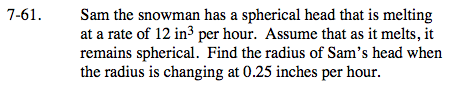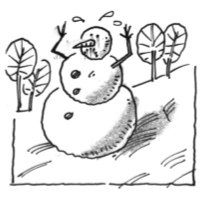### Home > CALC > Chapter 7 > Lesson 7.2.1 > Problem7-61

7-61.$V=\frac{4}{3}\pi r^{3}$

How can you construct an equation that describes how the volume of Sam's head is changing over time?

$\frac{dV}{dt}=-12, \ \ \ \frac{dr}{dt}=-0.25$r ≈ 1.954 _____
Be mindful of units.